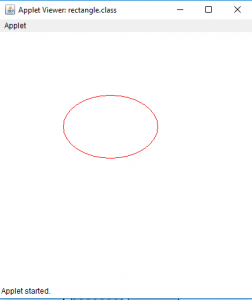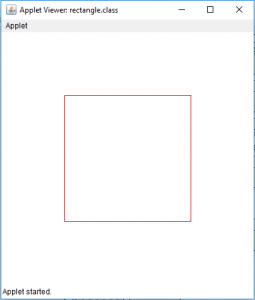GeeksforGeeks App
Open AppBrowser
Continue

# Draw a ellipse and a rectangle in Java Applet

Java applets are application that can be executed in web browsers or applet viewers . We can draw shapes on the Java applet. In this article we will draw a ellipse on Java applet by two ways . By using the drawOval(int x, int y, int width, int height) or by using mathematical formula (X= A * sin a, Y= B *cos a, where A and B are major and minor axes and a is the angle ) . Similarly, we will draw a rectangle on Java applet by two ways . By using the drawRect(int x, int y, int width, int height) or by drawing four lines joining the edges .

Note:
Java applets are deprecated, which means that it’s no longer recommended for use, and may be removed in future versions of Java. Applets are small programs that can be run inside a web page and in the past, it was popular technology but over time, its popularity decreased. Applets are considered as a security risk as they can execute arbitrary code on the client machine, and many browsers now disable them by default. Therefore, it’s not recommended to use Java applets for new projects, instead, there are other technologies like web-based applications or JavaScript, that are more secure and widely used. If your project requires applets technology, it’s better to consult experts and seek alternatives. It’s important to note that Java applets are still supported in current versions of Java, but it’s not a good idea to use it for new projects.

To draw a ellipse in Java Applet

Examples: Let us draw a oval with width 150 and height 100

```Input : x and y coordinates 100, 100 respectively
Width and height 150 and 100 respectively ```

Output :To draw a rectangle in Java Applet

Examples: We will draw a rectangle of height 200 and width 200 and At a position 100,100 on the applet.

```Input : x and y coordinates 100, 100 respectively
Width and height 200 and 200 respectively.```

Output :1. Java Program to draw a ellipse using drawOval(int x, int y, int width, int height)

## Java

 `// java program to draw a ellipse``// using drawOval function.``import` `java.awt.*;``import` `javax.swing.*;` `public` `class` `ellipse ``extends` `JApplet {` `    ``public` `void` `init()``    ``{``        ``// set size``        ``setSize(``400``, ``400``);` `        ``repaint();``    ``}` `    ``// paint the applet``    ``public` `void` `paint(Graphics g)``    ``{``        ``// set Color for rectangle``        ``g.setColor(Color.red);` `        ``// draw a ellipse``        ``g.drawOval(``100``, ``100``, ``150``, ``100``);``    ``}``}`

Output :2. program to draw a ellipse using drawLine function

## Java

 `// java program to draw a ellipse``// using drawLine function``import` `java.awt.*;``import` `javax.swing.*;` `public` `class` `ellipse ``extends` `JApplet {` `    ``public` `void` `init()``    ``{``        ``setSize(``300``, ``300``);``    ``}` `    ``public` `void` `paint(Graphics g)``    ``{``        ``// center of the``        ``int` `cx, cy;` `        ``// center of the ellipse``        ``cx = ``150``;``        ``cy = ``175``;` `        ``// major and minor axis``        ``double` `A = ``75``, B = ``50``, px = ``0``, py = ``0``;` `        ``// set color``        ``g.setColor(Color.red);` `        ``// draw the ellipse``        ``for` `(``int` `i = ``0``; i <= ``360``; i++) {``            ``double` `x, y;``            ``x = A * Math.sin(Math.toRadians(i));``            ``y = B * Math.cos(Math.toRadians(i));` `            ``if` `(i != ``0``) {``                ``// draw a line joining previous and new point .``                ``g.drawLine((``int``)px + cx, (``int``)py + cy,``                                ``(``int``)x + cx, (``int``)y + cy);``            ``}` `            ``// store the previous points``            ``px = x;``            ``py = y;``        ``}``    ``}``}`

Output :Now we will see how to draw a rectangle in a Java Applet We can draw rectangle in java applet by two ways.

1. Draw a rectangle using drawRect(int x, int y, int width, int height)

## Java

 `// Java Program to  Draw a rectangle``// using drawRect(int x, int y, int width, int height)``import` `java.awt.*;``import` `javax.swing.*;` `public` `class` `rectangle ``extends` `JApplet {` `    ``public` `void` `init()``    ``{``        ``// set size``        ``setSize(``400``, ``400``);` `        ``repaint();``    ``}` `    ``// paint the applet``    ``public` `void` `paint(Graphics g)``    ``{``        ``// set Color for rectangle``        ``g.setColor(Color.red);` `        ``// draw a rectangle``        ``g.drawRect(``100``, ``100``, ``200``, ``200``);``    ``}``}`

Output:2. Draw a rectangle using drawLine(int x, int y, int x1, int y1)

## Java

 `// Java Program  Draw a rectangle``// using drawLine(int x, int y, int x1, int y1)``import` `java.awt.*;``import` `javax.swing.*;` `public` `class` `rectangle ``extends` `JApplet {` `    ``public` `void` `init()``    ``{``        ``// set size``        ``setSize(``400``, ``400``);` `        ``repaint();``    ``}` `    ``// paint the applet``    ``public` `void` `paint(Graphics g)``    ``{``        ``// set Color for rectangle``        ``g.setColor(Color.red);` `        ``// draw a rectangle by drawing four lines``        ``g.drawLine(``100``, ``100``, ``100``, ``300``);``        ``g.drawLine(``100``, ``300``, ``300``, ``300``);``        ``g.drawLine(``300``, ``300``, ``300``, ``100``);``        ``g.drawLine(``300``, ``100``, ``100``, ``100``);``    ``}``}`

Output :Note : the following programs might not run in an online compiler please use an offline IDE.

My Personal Notes arrow_drop_up
Related Tutorials